# Reflection (geometry)

In Euclidean geometry, a reflection is a linear operation σ on ℝ3 with the property σ2 = E, the identity map. This property of σ is called involution. An involution is non-singular and is equal to its inverse: σ−1 = σ. Reflecting twice an arbitrary vector brings back the original vector: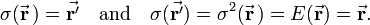$\sigma( \vec{\mathbf{r}}\,) = \vec{\mathbf{r'}} \quad\hbox{and}\quad \sigma( \vec{\mathbf{r'}}) = \sigma^2({\vec\mathbf{r}}\,) = E(\vec{\mathbf{r}}) = \vec{\mathbf{r}}.$

The operation σ is an isometry of ℝ3 onto itself, which means that it preserves inner products and hence that its inverse is equal to its adjoint,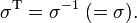$\sigma^\mathrm{T} = \sigma^{-1}\; ( = \sigma). \,$

It follows that reflection is symmetric: σT = σ. From the properties of determinants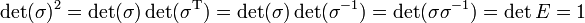$\det(\sigma)^2 = \det(\sigma)\det(\sigma^\mathrm{T}) = \det(\sigma)\det(\sigma^{-1}) = \det(\sigma\sigma^{-1}) = \det{E} = 1\,$

follows that isometries have det(σ) = ±1. Those with determinant +1 are rotations; those with determinant −1 are reflections.

A reflection σ on ℝ3 has two sets of eigenvalues: {1, 1, −1} and {−1, −1, −1}. This follows because the eigenvalues of σ2 = E are +1 and hence the eigenvalues of σ are ±1. The product of the eigenvalues being the determinant −1, the sets of eigenvalues of σ are either {1, 1, −1}, or {−1, −1, −1}. An operator with the latter set of eigenvalues is equal to −E, minus the identity operator. This operator is known alternatively as inversion, reflection in a point, or parity operator. An operator with the former set of eigenvalues is reflection in a plane. Reflections in a plane are the subject of this article.

Sometimes one finds the concept of "reflections in a line", these are rotations over 180°, see rotation matrix.Fig. 1. The vector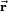$\vec{\mathbf{r}}$ goes to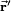$\scriptstyle\vec{\mathbf{r}}'$ under reflection in a plane. The unit vector$\scriptstyle\hat{\mathbf{n}}$ is normal to the mirror plane.

## Reflection in a plane

If$\hat{\mathbf{n}}$ is a unit vector normal (perpendicular) to a plane—the mirror plane—then$(\hat{\mathbf{n}}\cdot\vec{\mathbf{r}})\hat{\mathbf{n}}$ is the projection of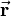$\vec{\mathbf{r}}$ on this unit vector. From the figure it is evident that$\vec{\mathbf{r}} - \vec{\mathbf{r}}\,' = 2 (\hat{\mathbf{n}}\cdot\vec{\mathbf{r}})\, \hat{\mathbf{n}} \;\Longrightarrow\; \vec{\mathbf{r}}\,' = \vec{\mathbf{r}} - 2 (\hat{\mathbf{n}}\cdot\vec{\mathbf{r}})\hat{\mathbf{n}}$

If a non-unit normal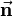$\vec{\mathbf{n}}$ is used then substitution of$\hat{\mathbf{n}} = \frac{\vec{\mathbf{n}}}{ |\vec{\mathbf{n}}|} \equiv \frac{\vec{\mathbf{n}}}{n}$

gives the mirror image,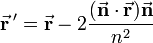$\vec{\mathbf{r}}\,' = \vec{\mathbf{r}} - 2 \frac{ (\vec{\mathbf{n}}\cdot\vec{\mathbf{r}})\vec{\mathbf{n}}}{n^2}$

Sometimes it is convenient to write this as a matrix equation. Introducing the dyadic product, we obtain$\vec{\mathbf{r}}\,' = \left[ \mathbf{E} - \frac{2}{n^2} \vec{\mathbf{n}} \otimes\vec{\mathbf{n}} \right] \; \vec{\mathbf{r}},$

where E is the 3×3 identity matrix.

Dyadic products satisfy the matrix multiplication rule$[\vec{\mathbf{a}}\otimes\vec{\mathbf{b}}]\, [ \vec{\mathbf{c}}\otimes\vec{\mathbf{d}}] = (\vec{\mathbf{b}} \cdot \vec{\mathbf{c}}) \big( \vec{\mathbf{a}}\otimes\vec{\mathbf{d}} \big).$

By the use of this rule it is easily shown that$\left[ \mathbf{E} - \frac{2}{n^2} \vec{\mathbf{n}} \otimes\vec{\mathbf{n}} \right]^2 = \mathbf{E},$

which confirms that reflection is involutory.Fig. 2. The vector$\vec{\mathbf{s}}$ goes to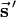$\vec{\mathbf{s}}\,'$ under reflection

## Reflection in a plane not through the origin

In Figure 2 a plane, not containing the origin O, is considered that is orthogonal to the vector$\vec{\mathbf{t}}$. The length of this vector is the distance from O to the plane. From Figure 2, we find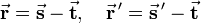$\vec{\mathbf{r}} = \vec{\mathbf{s}} - \vec{\mathbf{t}}, \quad \vec{\mathbf{r}}\,' = \vec{\mathbf{s}}\,' - \vec{\mathbf{t}}$

Use of the equation derived earlier gives$\vec{\mathbf{s}}\,' - \vec{\mathbf{t}} = \vec{\mathbf{s}} - \vec{\mathbf{t}} - 2 \big(\hat{\mathbf{n}}\cdot (\vec{\mathbf{s}} - \vec{\mathbf{t}})\big)\hat{\mathbf{n}}.$

And hence the equation for the reflected pair of vectors is,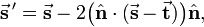$\vec{\mathbf{s}}\,' = \vec{\mathbf{s}} - 2 \big(\hat{\mathbf{n}}\cdot (\vec{\mathbf{s}} - \vec{\mathbf{t}})\big)\hat{\mathbf{n}},$

where$\hat{\mathbf{n}}$ is a unit vector normal to the plane. Obviously$\vec{\mathbf{t}}$ and$\hat{\mathbf{n}}$ are proportional, they differ only by scaling. Therefore, the equation can be written solely in terms of$\vec{\mathbf{t}}$,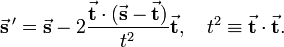$\vec{\mathbf{s}}\,' = \vec{\mathbf{s}} - 2 \frac{\vec{\mathbf{t}}\cdot (\vec{\mathbf{s}} - \vec{\mathbf{t}})}{t^2}\vec{\mathbf{t}}, \quad t^2 \equiv \vec{\mathbf{t}}\cdot \vec{\mathbf{t}}.$

## Two consecutive reflections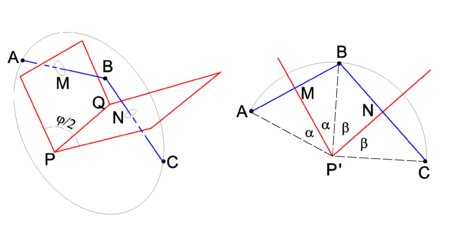Fig. 3. Two reflections. Left drawing: 3-dimensional drawing. Right drawing: view along the PQ axis, drawing projected on the plane through ABC. This plane intersect the line PQ in the point P′

Two consecutive reflections in two intersecting planes give a rotation around the line of intersection. This is shown in Figure 3, where PQ is the line of intersection. The drawing on the left shows that reflection of point A in the plane through PMQ brings the point A to B. A consecutive reflection in the plane through PNQ brings B to the final position C. In the right-hand drawing it is shown that the rotation angle φ is equal to twice the angle between the mirror planes. Indeed, the angle ∠ AP'M = ∠ MP'B = α and ∠ BP'N = ∠ NP'C = β. The rotation angle ∠ AP'C ≡ φ = 2α + 2β and the angle between the planes is α+β = φ/2.

It is obvious that the product of two reflections is a rotation. Indeed, a reflection is an isometry and has determinant −1. The product of two isometric operators is again an isometry and the rule for determinants is det(AB) = det(A)det(B), so that the product of two reflections is an isometry with unit determinant, i.e., a rotation.

Let the normal of the first plane be$\vec{\mathbf{s}}$ and of the second$\vec{\mathbf{t}}$, then the rotation is represented by the matrix$\left[ \mathbf{E} - \frac{2}{t^2} \vec{\mathbf{t}} \otimes\vec{\mathbf{t}} \right]\, \left[ \mathbf{E} - \frac{2}{s^2} \vec{\mathbf{s}} \otimes\vec{\mathbf{s}} \right] = \mathbf{E} - \frac{2}{t^2} \vec{\mathbf{t}} \otimes\vec{\mathbf{t}} - \frac{2}{s^2} \vec{\mathbf{s}} \otimes\vec{\mathbf{s}} + \frac{4}{t^2 s^2} (\vec{\mathbf{t}}\cdot\vec{\mathbf{s}})\; \big(\vec{\mathbf{t}} \otimes\vec{\mathbf{s}}\big)$

The (i,j) element if this matrix is equal to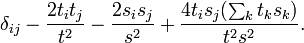$\delta_{ij} - \frac{2 t_i t_j }{t^2} - \frac{2 s_i s_j }{s^2} + \frac{4 t_i s_j (\sum_k t_k s_k)}{t^2 s^2} .$

This formula is used in vector rotation.